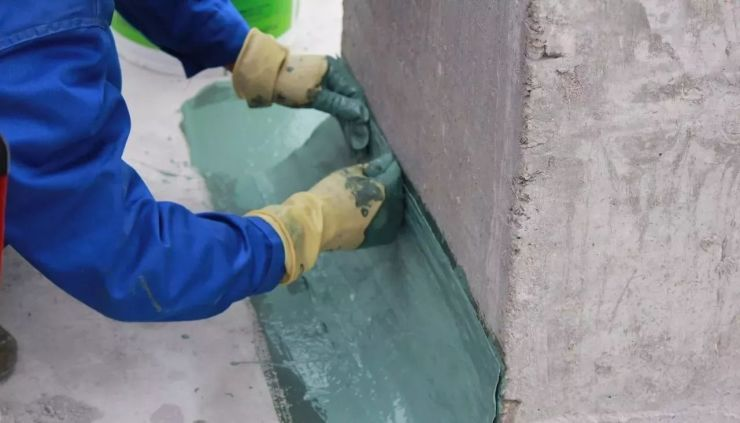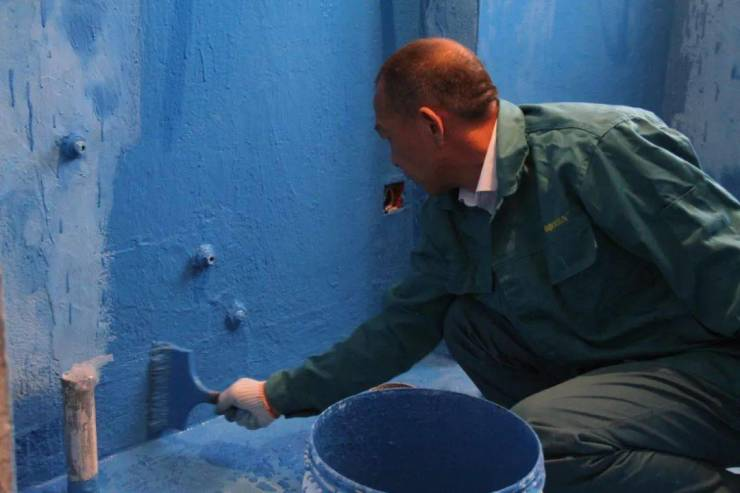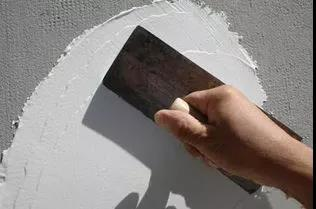|

# 建筑防水涂料的施工工艺

1、刷涂（1）刷涂的基本操作方法（2）刷涂的注意事项

①刷涂时漆刷蘸涂料、涂布、抹平、修整这几个步骤应该是连贯的，不应该有停顿的间隙。熟练的操作者可以将这几个操作步骤一步完成。

②涂布、抹平、修整三个步骤应纵横交替地刷涂，但被涂物的垂直面，最后一个步骤应沿着垂直方向进行竖刷。刷涂木质被涂物时最后一个步骤应与木纹方向相同。

③在进行涂布和抹平操作时，漆刷要求处于垂直状态，并用力将刷毛大部分贴附在被涂物表面。但在修整时，漆刷应向运行的方向倾斜，用刷毛的前端轻轻地刷涂修整，以便达到满意的修整效果。

④漆刷每次的涂料蘸量最好基本保持一致。只要漆刷的规格选用得当，漆刷每次蘸的涂料刷涂面积也能基本保持一致。

⑤刷涂面积较大的被涂物时，通常应先从左上角开始刷涂，每蘸一次涂料后按照涂布、抹平、修整三个步骤完成一块刷涂面积后，再蘸涂料刷涂下一块刷涂面积。

⑥仰面刷涂时，漆刷涂料要少一点，刷涂时用力不要太重，漆刷运行不要太快，以免涂料掉落。2、刮涂

（1）腻子的刮涂

①腻子刮涂的次数。腻子要进行多次刮涂，腻子层才能牢固结实，不能要求一次刮涂的腻子层即达到预定的厚度。因为一次刮涂过厚，腻子层容易开裂脱落，且干燥慢。为保证刮涂质量，一般刮涂不少于三次，即通常所说的头道、二道、末道。对三次刮涂各自的要求是不相同的：

②腻子稠度的调整。腻子稠度与刮涂效果有密切的关系，稠度适当才能浸润底层又能确保必要的厚度。腻子稠度通常随时间而增高，这是稀释剂挥发的结果。在刮涂前如发现腻子的稠度过高，不符合刮涂要求，应用与其配套的稀释剂进行调整。

③刮涂腻子的步骤。刮涂操作通常分为抹涂、刮平、修整三个步骤，但要根据刮涂的要求灵活运用。干燥速度慢的腻子与干燥速度快的腻子刮涂时的三个步骤是有区别的，前者可以明显地分为三个步骤，后者如过氯乙烯树脂腻子干燥速度快，刮涂时不能明显地分为三个步骤，抹涂、刮平、修整应连续一步完成。a.抹涂是用刮刀将腻子抹涂在被涂物表面，抹涂时先用刮刀从腻子托盘中挖取腻子，然后将刮刀的刃口贴附在被涂物的表面，刮刀运行初期应稍向前倾斜，与被涂物表面呈80°夹角，随着刮刀运行移动，腻子不断地转移到被涂物表面，同时刮刀上的腻子逐渐减少，因此要求刮刀在移动过程中逐渐加大向前倾斜的程度，迫使腻子黏附在被涂物表面，直至夹角约为30°时，将刮刀黏附的腻子完全抹涂在被涂物表面。

b.刮平是将抹涂在被涂物表面的腻子层刮涂平整，消除明显的抹涂痕迹，刮平时应先将刮刀上残留的腻子去掉，然后用力将刮刀尽量向前倾斜贴附于腻子层上，并按照抹涂时刮刀的运行轨迹向前刮，随着刮刀的移动，刮刀上黏附的腻子会逐渐增多，刮刀与被涂物表面的夹角也应逐渐增大，直到夹角呈90°时把多余的腻子刮下来。

c.修整是腻子层已基本刮涂平整后，修整个别不平整的缺陷、接缝痕迹、边沿缺损等。修整时刮刀应向前倾斜，或用少许腻子填补，或用刮刀挤刮，用力不宜过大，以防损坏整个腻子层。

④打磨腻子。刮涂的腻子层往往表面粗糙，留有刮痕及其他缺陷，需要打磨才能达到平整光滑的要求，打磨腻子是刮涂所必需的后处理工序。

⑤刮涂的注意事项

a.选用的腻子要与整个涂装体系配套，即与底漆、面漆配套，为调整黏度除可以添加配套的稀释剂外，不能在腻子中间添加任何其他的填料；

b.被涂物表面应清理干净，如发现原涂底漆漆膜脱落或出现锈蚀时，应重新进行表面处理；

c.要根据被涂物的表面形状与刮涂要求，正确选用刮刀；

d.刮涂一个被涂物时，其操作顺序应先上后下，先左后右，先平面后棱角；

e.每道腻子都不能刮得过厚，要刮得结实，但不能漏刮，不能有气泡，厚度最好控制在0.3~0.5mm范围内，二道腻子也不应超过1mm，腻子层必须干结后，方可进行下一道刮涂或打磨；

f.刮刀在使用过程中难免会有损伤，要及时进行修整，使刀刃保持垂直；

g.采用湿磨方法打磨时，为防止钢铁被涂物锈蚀，最好采用防锈打磨，防锈水可参考如下配方：硼纱1%(质量分数)、三乙醇胺0.2%(质量分数)、香精0.003%(质量分数)、水适量。（2）防水涂料的刮涂

①刮涂施工工艺。刮涂常用的工具有牛角刀、油灰刀、橡皮刮刀和钢皮刮刀等。

a.刮涂时应用刮刀，使刮刀与被涂面的倾斜角为50°~60°，刮刀要用力均匀。

b.对涂层厚度的控制采用预先在刮板上固定铁丝(或木条)或在屋面上做好标志的方法。铁丝(或木条)的高度应与每遍涂层厚度要求一致。一般需刮涂两至三遍，总厚度为4~8mm。

c.刮涂时只能来回刮1~2次，不能往返多次刮涂，否则将会出现“皮干里不干”现象。

d.遇有圆、棱形基面，可用橡皮刮刀进行刮涂。

e.为了加快施工进度，可采用分条间隔施工，待先批涂层干燥后，再抹后批空白处。分条宽度一般为0.8~1.0m，以便于抹压操作，并与胎体增强材料宽度相一致。

f.待前一遍涂料完全干燥后可进行下一遍涂料施工。一般以脚踩不沾脚、不下陷(或下陷能回弹)时才进行下一道涂层施工，干燥时间不宜少于12h。

g.当涂膜出现气泡、皱褶不平、凹陷、刮痕等情况，应立即进行修补。修补好后才能进行下一道涂膜施工。

②刮涂施工注意事项

a.防水涂料使用前应特别注意搅拌均匀，因为厚质防水涂料内有较多的填充料，如搅拌不均匀，不仅涂刮困难，而且未搅匀的颗粒杂质残留在涂层中，将成为隐患。

b.为了增加防水层与基层的结合力，可在基层上先涂刷一遍基层处理剂。若使用某些渗透力强的涂水防料，可不涂刷基层处理剂。

c.防水涂料的稠度一般应根据施工条件、厚度要求等因素确定。

d.待前一遍涂料完全干燥，缺陷修补完毕并干燥后，才能进行下一遍涂料施工。后一遍涂料的刮涂方向应与前一遍刮涂方向垂直。

e.立面部位涂层应在平面涂层施工前进行，视涂料的流平性好坏确定涂刮遍数。流平性好的涂料应按照多遍薄刮的原则进行，以免产生流坠现象，使上部涂层变薄，下部涂层变厚，影响防水质量。

f.防水涂层施工完毕，应注意养护和成品保护。

③刮涂施工质量要求

`声明：本文由入驻焦点开放平台的作者撰写，除焦点官方账号外，观点仅代表作者本人，不代表焦点立场错误信息举报电话： 400-099-0099，邮箱：jubao@vip.sohu.com，或点此进行意见反馈，或点此进行举报投诉。`A B C D E F G H J K L M N P Q R S T W X Y Z
A - B - C - D - E
• A
• 鞍山
• 安庆
• 安阳
• 安顺
• 安康
• 澳门
• B
• 北京
• 保定
• 包头
• 巴彦淖尔
• 本溪
• 蚌埠
• 亳州
• 滨州
• 北海
• 百色
• 巴中
• 毕节
• 保山
• 宝鸡
• 白银
• 巴州
• C
• 承德
• 沧州
• 长治
• 赤峰
• 朝阳
• 长春
• 常州
• 滁州
• 池州
• 长沙
• 常德
• 郴州
• 潮州
• 崇左
• 重庆
• 成都
• 楚雄
• 昌都
• 慈溪
• 常熟
• D
• 大同
• 大连
• 丹东
• 大庆
• 东营
• 德州
• 东莞
• 德阳
• 达州
• 大理
• 德宏
• 定西
• 儋州
• 东平
• E
• 鄂尔多斯
• 鄂州
• 恩施
F - G - H - I - J
• F
• 抚顺
• 阜新
• 阜阳
• 福州
• 抚州
• 佛山
• 防城港
• G
• 赣州
• 广州
• 桂林
• 贵港
• 广元
• 广安
• 贵阳
• 固原
• H
• 邯郸
• 衡水
• 呼和浩特
• 呼伦贝尔
• 葫芦岛
• 哈尔滨
• 黑河
• 淮安
• 杭州
• 湖州
• 合肥
• 淮南
• 淮北
• 黄山
• 菏泽
• 鹤壁
• 黄石
• 黄冈
• 衡阳
• 怀化
• 惠州
• 河源
• 贺州
• 河池
• 海口
• 红河
• 汉中
• 海东
• 怀来
• I
• J
• 晋中
• 锦州
• 吉林
• 鸡西
• 佳木斯
• 嘉兴
• 金华
• 景德镇
• 九江
• 吉安
• 济南
• 济宁
• 焦作
• 荆门
• 荆州
• 江门
• 揭阳
• 金昌
• 酒泉
• 嘉峪关
K - L - M - N - P
• K
• 开封
• 昆明
• 昆山
• L
• 廊坊
• 临汾
• 辽阳
• 连云港
• 丽水
• 六安
• 龙岩
• 莱芜
• 临沂
• 聊城
• 洛阳
• 漯河
• 娄底
• 柳州
• 来宾
• 泸州
• 乐山
• 六盘水
• 丽江
• 临沧
• 拉萨
• 林芝
• 兰州
• 陇南
• M
• 牡丹江
• 马鞍山
• 茂名
• 梅州
• 绵阳
• 眉山
• N
• 南京
• 南通
• 宁波
• 南平
• 宁德
• 南昌
• 南阳
• 南宁
• 内江
• 南充
• P
• 盘锦
• 莆田
• 平顶山
• 濮阳
• 攀枝花
• 普洱
• 平凉
Q - R - S - T - W
• Q
• 秦皇岛
• 齐齐哈尔
• 衢州
• 泉州
• 青岛
• 清远
• 钦州
• 黔南
• 曲靖
• 庆阳
• R
• 日照
• 日喀则
• S
• 石家庄
• 沈阳
• 双鸭山
• 绥化
• 上海
• 苏州
• 宿迁
• 绍兴
• 宿州
• 三明
• 上饶
• 三门峡
• 商丘
• 十堰
• 随州
• 邵阳
• 韶关
• 深圳
• 汕头
• 汕尾
• 三亚
• 三沙
• 遂宁
• 山南
• 商洛
• 石嘴山
• T
• 天津
• 唐山
• 太原
• 通辽
• 铁岭
• 泰州
• 台州
• 铜陵
• 泰安
• 铜仁
• 铜川
• 天水
• 天门
• W
• 乌海
• 乌兰察布
• 无锡
• 温州
• 芜湖
• 潍坊
• 威海
• 武汉
• 梧州
• 渭南
• 武威
• 吴忠
• 乌鲁木齐
X - Y - Z
• X
• 邢台
• 徐州
• 宣城
• 厦门
• 新乡
• 许昌
• 信阳
• 襄阳
• 孝感
• 咸宁
• 湘潭
• 湘西
• 西双版纳
• 西安
• 咸阳
• 西宁
• 仙桃
• 西昌
• Y
• 运城
• 营口
• 盐城
• 扬州
• 鹰潭
• 宜春
• 烟台
• 宜昌
• 岳阳
• 益阳
• 永州
• 阳江
• 云浮
• 玉林
• 宜宾
• 雅安
• 玉溪
• 延安
• 榆林
• 银川
• Z
• 张家口
• 镇江
• 舟山
• 漳州
• 淄博
• 枣庄
• 郑州
• 周口
• 驻马店
• 株洲
• 张家界
• 珠海
• 湛江
• 肇庆
• 中山
• 自贡
• 资阳
• 遵义
• 昭通
• 张掖
• 中卫

1室1厅1厨1卫1阳台

1
2
3
4
5

0
1
2

1

1

0
1
2
3报名成功，资料已提交审核A B C D E F G H J K L M N P Q R S T W X Y Z
A - B - C - D - E
• A
• 鞍山
• 安庆
• 安阳
• 安顺
• 安康
• 澳门
• B
• 北京
• 保定
• 包头
• 巴彦淖尔
• 本溪
• 蚌埠
• 亳州
• 滨州
• 北海
• 百色
• 巴中
• 毕节
• 保山
• 宝鸡
• 白银
• 巴州
• C
• 承德
• 沧州
• 长治
• 赤峰
• 朝阳
• 长春
• 常州
• 滁州
• 池州
• 长沙
• 常德
• 郴州
• 潮州
• 崇左
• 重庆
• 成都
• 楚雄
• 昌都
• 慈溪
• 常熟
• D
• 大同
• 大连
• 丹东
• 大庆
• 东营
• 德州
• 东莞
• 德阳
• 达州
• 大理
• 德宏
• 定西
• 儋州
• 东平
• E
• 鄂尔多斯
• 鄂州
• 恩施
F - G - H - I - J
• F
• 抚顺
• 阜新
• 阜阳
• 福州
• 抚州
• 佛山
• 防城港
• G
• 赣州
• 广州
• 桂林
• 贵港
• 广元
• 广安
• 贵阳
• 固原
• H
• 邯郸
• 衡水
• 呼和浩特
• 呼伦贝尔
• 葫芦岛
• 哈尔滨
• 黑河
• 淮安
• 杭州
• 湖州
• 合肥
• 淮南
• 淮北
• 黄山
• 菏泽
• 鹤壁
• 黄石
• 黄冈
• 衡阳
• 怀化
• 惠州
• 河源
• 贺州
• 河池
• 海口
• 红河
• 汉中
• 海东
• 怀来
• I
• J
• 晋中
• 锦州
• 吉林
• 鸡西
• 佳木斯
• 嘉兴
• 金华
• 景德镇
• 九江
• 吉安
• 济南
• 济宁
• 焦作
• 荆门
• 荆州
• 江门
• 揭阳
• 金昌
• 酒泉
• 嘉峪关
K - L - M - N - P
• K
• 开封
• 昆明
• 昆山
• L
• 廊坊
• 临汾
• 辽阳
• 连云港
• 丽水
• 六安
• 龙岩
• 莱芜
• 临沂
• 聊城
• 洛阳
• 漯河
• 娄底
• 柳州
• 来宾
• 泸州
• 乐山
• 六盘水
• 丽江
• 临沧
• 拉萨
• 林芝
• 兰州
• 陇南
• M
• 牡丹江
• 马鞍山
• 茂名
• 梅州
• 绵阳
• 眉山
• N
• 南京
• 南通
• 宁波
• 南平
• 宁德
• 南昌
• 南阳
• 南宁
• 内江
• 南充
• P
• 盘锦
• 莆田
• 平顶山
• 濮阳
• 攀枝花
• 普洱
• 平凉
Q - R - S - T - W
• Q
• 秦皇岛
• 齐齐哈尔
• 衢州
• 泉州
• 青岛
• 清远
• 钦州
• 黔南
• 曲靖
• 庆阳
• R
• 日照
• 日喀则
• S
• 石家庄
• 沈阳
• 双鸭山
• 绥化
• 上海
• 苏州
• 宿迁
• 绍兴
• 宿州
• 三明
• 上饶
• 三门峡
• 商丘
• 十堰
• 随州
• 邵阳
• 韶关
• 深圳
• 汕头
• 汕尾
• 三亚
• 三沙
• 遂宁
• 山南
• 商洛
• 石嘴山
• T
• 天津
• 唐山
• 太原
• 通辽
• 铁岭
• 泰州
• 台州
• 铜陵
• 泰安
• 铜仁
• 铜川
• 天水
• 天门
• W
• 乌海
• 乌兰察布
• 无锡
• 温州
• 芜湖
• 潍坊
• 威海
• 武汉
• 梧州
• 渭南
• 武威
• 吴忠
• 乌鲁木齐
X - Y - Z
• X
• 邢台
• 徐州
• 宣城
• 厦门
• 新乡
• 许昌
• 信阳
• 襄阳
• 孝感
• 咸宁
• 湘潭
• 湘西
• 西双版纳
• 西安
• 咸阳
• 西宁
• 仙桃
• 西昌
• Y
• 运城
• 营口
• 盐城
• 扬州
• 鹰潭
• 宜春
• 烟台
• 宜昌
• 岳阳
• 益阳
• 永州
• 阳江
• 云浮
• 玉林
• 宜宾
• 雅安
• 玉溪
• 延安
• 榆林
• 银川
• Z
• 张家口
• 镇江
• 舟山
• 漳州
• 淄博
• 枣庄
• 郑州
• 周口
• 驻马店
• 株洲
• 张家界
• 珠海
• 湛江
• 肇庆
• 中山
• 自贡
• 资阳
• 遵义
• 昭通
• 张掖
• 中卫• 手机• 分享
• 设计
免费设计
• 计算器
装修计算器
• 入驻
合作入驻
• 联系
联系我们
• 置顶
返回顶部